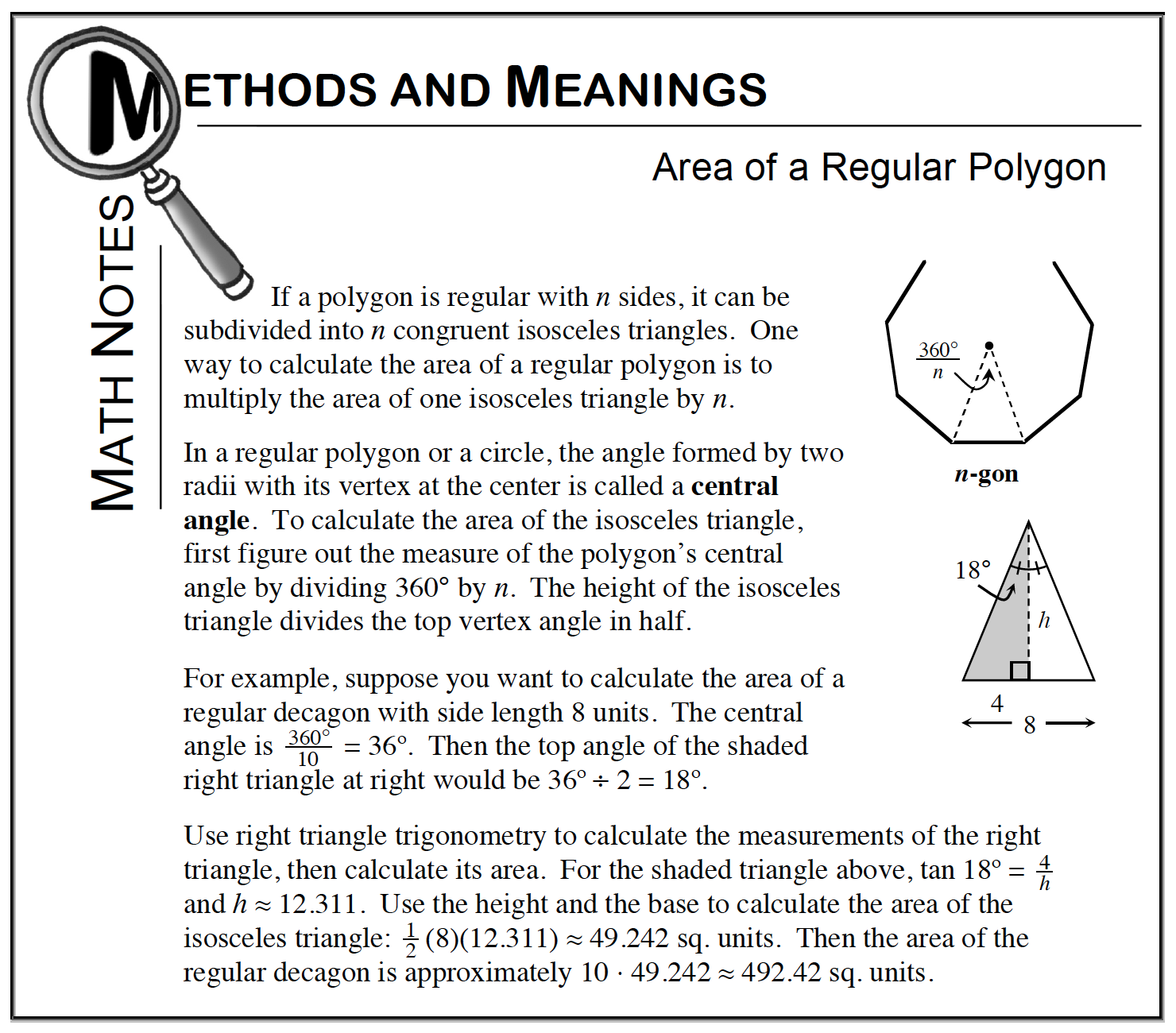### Home > INT2 > Chapter 8 > Lesson 8.3.1 > Problem8-59

8-59.

A regular hexagon with side length $4$ has the same area as a square. What is the length of the side of the square? Explain how you know. Homework Help ✎

Follow the steps outlined in the Math Notes box below to calculate the area of the hexagon.

The area of a square is $\text{A}=s^2$. So to determine the side length of the square take the square root of the hexagon's area (since they are equal).